# Single-Carrier FDMA and Filtered-OFDM¶

Now that we have a basic understanding of the CP-OFDM waveform and the required components to make it run, we can consider OFDM-related waveforms. This is in particular important, as the upcoming 5G standard has decided to abandon CP-based OFDM, but rely more on a filtered variant, named Filtered-OFDM (F-OFDM). In this notebook, we will have a short look onto this waveform. In addition, we will look at the waveform which is used in the 4G LTE uplink, named Single-carrier frequency division multiple access (SC-FDMA).

Two main drawbacks of CP-OFDM, which are addressed by SC-FDMA and F-OFDM are its high PAPR and bad spectral properties. We will have a look at both properties below. As these considerations are advanced compared to undergraduate studies, the treatment here is more applied than mathematical.

To start, let us first go ahead and implement transmitters for F-OFDM and SC-FDMA:

## Implementation of OFDM, SC-FDMA and F-OFDM¶

In :
from audioComms.buffers import PeriodicBuffer, RingBuffer
from audioComms.utils import StatefulFilter
from audioComms.components import Component, TX, Environment, Recorder
from audioComms.plotting import PlotWaveform, PlotSpectrum, PlotConstellation, PlotBlock, PlotPAPR, PlotAmplitudeCCDF, PlotPowerAndClippingRatio
from audioComms.channels import AudioChannel, SimulatedChannel
from audioComms.channels import IdentityChannelEffect, SimulatedChannelEffect, NoiseChannelEffect, ChainedChannelEffects, MultipathChannelEffect

from audioComms.passband import PassbandChannel, UpDownConversionFilter

In :
# Source code has been redacted in online version
# Omitting 6 lines of source code


For reference, let us create a very basic OFDM transmitter, which is used below to analyze the spectral confinement and PAPR of CP-OFDM. To recap, the OFDM modulation equation is given by:

$$x_\text{CP-OFDM}[n] = \text{IDFT}_K\{X[k]\}$$

where $X[k]$ are the QAM symbols in the frequency domain. In this notebook, we consider a non-full OFDM allocation, as we have also done before. This means, that the carriers with the highest frequencies are not allocated and hence transmit zero. In particular, we assume an allocation of $K_\text{on}$ continuous subcarriers.

In :
class OFDMTransmitter(TX):
def __init__(self, environment, ofdm, gain=1):
super().__init__(environment)
self._ofdm = ofdm
self._gain = gain

def _generateSignal(self):
qam = random_qam(self._ofdm)              # Create random data
sym = ofdm_modulate(self._ofdm, qam)      # Perform OFDM modulation
return sym*self._gain


### Single-carrier FDMA (SC-FDMA):¶

The single-carrier FDMA system can be understood as an OFDM transmitter with an additional precoding before OFDM modulation. The precoding equals an $K_\text{on}$-point DFT and hence the transmit signal before CP-insertion is given by

$$x_\text{SC-FDMA}[n]=\text{IDFT}_K\{\text{DFT}_{K_\text{on}}\{X[k]\}\}$$

We can directly implement this equation as follows:

In :
# Source code has been redacted in online version
# Omitting 11 lines of source code


### Filtered-OFDM (F-OFDM)¶

The F-OFDM waveform arises from a standard CP-OFDM system with an addition filtering operation afterwards. Mathematically, it's as simple as

$$x_\text{F-OFDM}[n]=h[n] * x_\text{CP-OFDM}[n]$$

where in this case $x_\text{CP-OFDM}[n]$ is the continuous signal of the standard OFDM modulator, i.e. including the CP and all OFDM symbols follow after each other. $h[n]$ is the filter (sometimes called subband filter when the F-OFDM transmitter serves multiple service simultaneously). This filter does improve the spectral properties of the system, but can also introduce extra ISI if the filter is longer than the CP.

There are several filter designs available in the literature for the subband filter. Here, we resort to the initial proposal from https://arxiv.org/pdf/1508.07387.pdf ("Filtered-OFDM â€” Enabler for Flexible Waveform in The 5th Generation Cellular Networks" written by Xi Zhang, Ming Jia, Lei Chen, Jianglei Ma, Jing Qiu). There, the authors propose a hann-windowed sinc filter with a bandwidth of a bit wider than the allocated bandwidth (the guard carriers). where the overall filter length equals half the OFDM symbol length. Accordingly, let's design the filter as follows:

In :
def calcFOFDMFilter(ofdm):
L = ofdm.K // 2 + 1
n = np.arange(L+1)- L//2
guardCarriers = 2
pb = np.sinc(n*(ofdm.Kon+guardCarriers)/ofdm.K)
w = (0.5*(1+np.cos(2*np.pi*n/(L-1))))**0.5;

b = pb * w; b = b / np.sqrt(sum(abs(b)**2))
return StatefulFilter(b, np.array())


Let's have a look at this filter design:

In :
ofdm_for_filter = OFDM(K=256, Kon=100, CP=16, Npayload=2, qam=16)
F = calcFOFDMFilter(ofdm_for_filter)
h = F._b  # obtain the filter coefficients

plt.figure(figsize=(10,3));
plt.subplot(121); plt.title('Filter impulse response')
t = np.arange(len(h)) / ofdm_for_filter.K
plt.plot(t, h); plt.grid(True); plt.xlabel('n/K'); plt.ylabel('$h[n]$');

plt.subplot(122); plt.title('Filter frequency response')
H = np.fft.fft(h, 4*len(h))
f = np.linspace(-ofdm_for_filter.K/2, ofdm_for_filter.K/2, len(H), endpoint=False)
plt.plot(f, 20*np.log10(np.fft.fftshift(abs(H))));
plt.grid(True); plt.xlabel('f [Subcarriers]'); plt.ylabel('$|H[f]|$ [dB]'); plt.xlim((-ofdm_for_filter.K/2, ofdm_for_filter.K/2))
plt.tight_layout()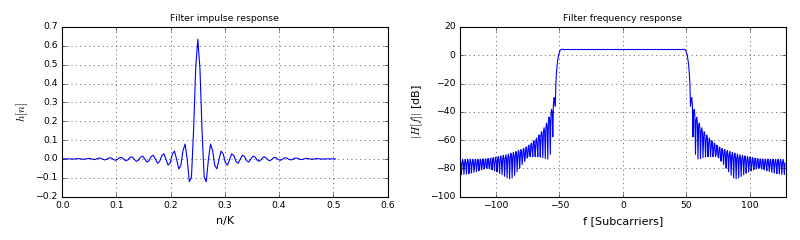You can play around with the parameter ofdm_for_filter.Kon parameter to change the bandwidth of the filter and hence the impulse and frequency response. We see that the filter has a steep transition region in the frequency domain, which is important to keep the signal spectrum well confined.

With this filter design, we can go ahead and implement the F-OFDM transmitter. It becomes as straight-forward as

In :
# Source code has been redacted in online version
# Omitting 18 lines of source code


Now that we have implemented CP-OFDM, SC-FDMA and F-OFDM transmitters, let us look at the mentioned properties of spectral confinement and PAPR. Let's start with the spectral properties:

## Out-of-band emission of CP-OFDM and F-OFDM¶

One complaint about CP-OFDM is its high out-of-band (OOB) radiation. Plainly, this means that a lot of energy is contained within frequencies that are not part of the allocated OFDM carriers. The high OOB emission of OFDM is due to the abrupt signal changes between OFDM blocks: The starting value of one OFDM symbol can be completely different from the ending value of the previous symbol. These signal jumps create high-frequency components, which reflect as OOB emission in the signal. More mathematically, we can understand that each OFDM symbol is windowed with a rectangular window and several symbols are concatenated after each other. This windowing operation corresponds to a convolution with the Fourier Transform of the window in the frequency domain. As the window is rectangular, the frequency domain equivalent is a sinc function, which decays very slowly.

Let us look at the spectrum of the OFDM signal and its filtered variant F-OFDM. First, let's consider the baseband signal:

In :
# Create OFDM and F-OFDM Transmitter objects
ofdm_for_spectrum = OFDM(K=256, Kon=100, CP=16, qam=4)
ofdmTX = OFDMTransmitter(None, ofdm_for_spectrum)
fofdmTX = FOFDMTransmitter(None, ofdm_for_spectrum)

L = 256  # number of blocks to concatenate
# Create signal blocks and concatenate them
ofdm_signal = np.hstack([ofdmTX._generateSignal() for _ in range(L)])
fofdm_signal = np.hstack([fofdmTX._generateSignal() for _ in range(L)])

# calculate spectrum
spec_ofdm = np.fft.fft(ofdm_signal) / np.sqrt(len(ofdm_signal))
spec_fofdm = np.fft.fft(fofdm_signal) / np.sqrt(len(fofdm_signal))

# plot the spectrum
f_ofdm = np.linspace(-ofdm_for_spectrum.K/2, ofdm_for_spectrum.K/2, len(spec_ofdm), endpoint=False)
f_fofdm = np.linspace(-ofdm_for_spectrum.K/2, ofdm_for_spectrum.K/2, len(spec_fofdm), endpoint=False)
plt.figure(figsize=(10,3)); plt.title('Baseband spetrum')
plt.plot(f_ofdm, np.fft.fftshift(20*np.log10(abs(spec_ofdm))), label='OFDM')
plt.plot(f_ofdm, np.fft.fftshift(20*np.log10(abs(spec_fofdm))), 'r', lw=2, label='F-OFDM', alpha=0.5)
plt.grid(True); plt.legend(loc='best'); plt.xlim((f_ofdm.min(), f_ofdm.max())); plt.xlabel('f [subcarriers]'); plt.ylabel('$X(f)$');
plt.gca().add_patch(patches.Rectangle(xy=(-110, -100), width=40, height=200, fill='red', color='green', alpha=0.3))
plt.tight_layout()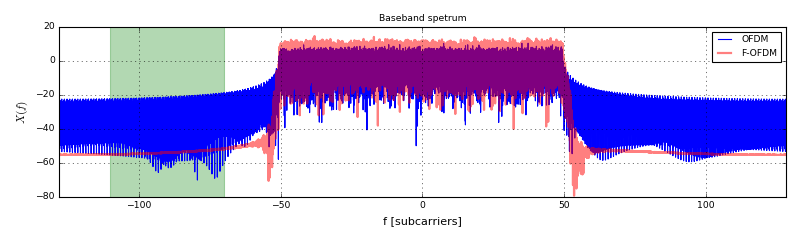Indeed, the raw OFDM signal (in blue) reaches the spectrum floor at -23dB, whereas the F-OFDM variant achieves a spectrum floor of -50dB. Clearly, if another system was supposed to operate in the marked green region, it would receive much more interference from the blue system (CP-OFDM) compared to F-OFDM. In fact, this property is the motivation for employing F-OFDM in 5G.

Now, that we have seen the difference in the baseband, let's look at what actually happens with the real transmitted RF signal. Below, we define a function to transmit OFDM or F-OFDM signals, using different DA filter settings. In addition, the function marks different OOB regions: the dashed region is governed by the actual waveform, this region is within the baseband bandwidth. The dotted region describes the spectral areas that are influenced by the digital-to-analog interpolation filter.

In :
# Source code has been redacted in online version
# Omitting 32 lines of source code

In :
ofdm = OFDM(K=256, Kon=201, CP=16, Npayload=2, qam=16)


First, let's look at the spectrum with the default OFDM transmitter:

In :
showTXSpectrum(ofdm, OFDMTransmitter)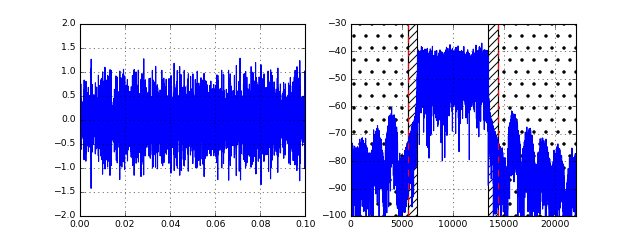Stop received from external


What happens? We see significant peaks within the dotted region. Let us see, if the cause is the waveform or the DA-filter. For comparison, we run the same function, but this time using the F-OFDM transmitter:

In :
showTXSpectrum(ofdm, FOFDMTransmitter)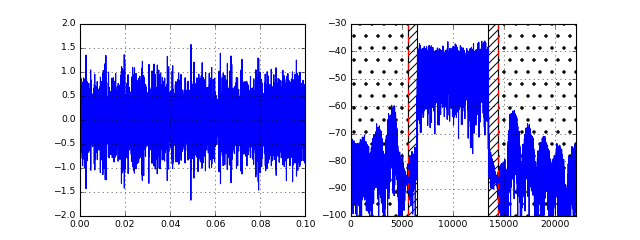Stop received from external


Hm, even though we are sure the waveform itself creates a smooth spectrum, we still see the very high sidepeaks in the overall spectrum. If we look closely, within the baseband bandwidth (dashed area), the spectrum is much better than that of the OFDM signal. However, within the dotted region, both signal exhibit the same spectral distribution.

As we have seen in the notebook about filter design for up-down-conversion, the spectral performance strongly depends on the digital-to-analog conversion filter. Let's tune this filter and see what happens. First, let's add a window around the DA filter:

In :
showTXSpectrum(ofdm, FOFDMTransmitter, AD_DA_filterUseWindow=True)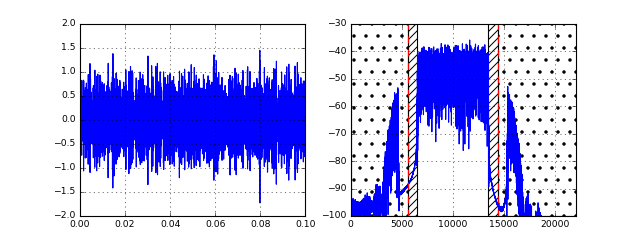Stop received from external


Indeed, the spectrum improves in general, however the height of the first side lobe even increases. We can explain this with our observations from the filter design notebook, where we saw that the filter achieves a higher attenuation but also becomes wider when employing a window.

Now, let's proceed and in addition to windowing the filter, let's increase its length such that it more closely approximates an infinitely long filter:

In :
showTXSpectrum(ofdm, FOFDMTransmitter, AD_DA_filterUseWindow=True, AD_DA_filterLength=9)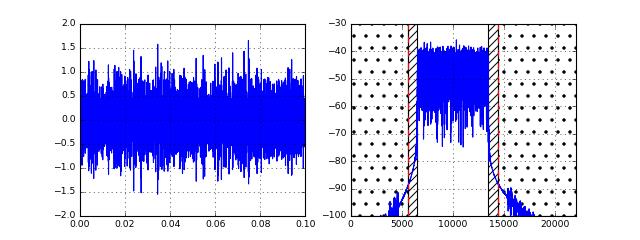Stop received from external


Ah! Great, now the spectrum looks much better. Still, we see some artifacts in the dotted region, but in general the spectrum is fine now. Let's compare this F-OFDM spectrum with the plain CP-OFDM spectrum:

In :
showTXSpectrum(ofdm, OFDMTransmitter, AD_DA_filterUseWindow=True, AD_DA_filterLength=9)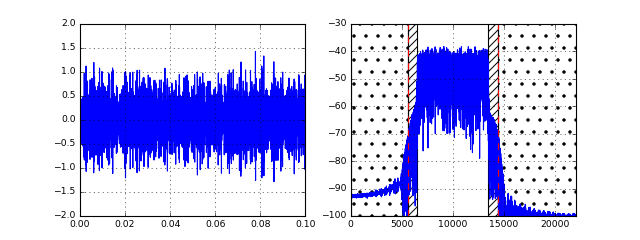Stop received from external


We see a similar spectral performance in the dotted region (i.e. the DA-filter governed region). However, within the dashed region (the baseband bandwidth) the spectrum of CP-OFDM is much higher compared to F-OFDM, introducing more interference to possibly spectrally adjacent system.

Now, to emphasize our findings, let us consider a system that has a much narrower allocation (i.e. less carriers are used). Such configuration is used when the baseband sample rate is constant, but our system would have to flexibly adapt to different bandwidth requirements.

Moreover, a non-full allocation also happens in 4G LTE: The clock rate and hence baseband bandwith is 30.72MHz, however the channel bandwidth is only 20MHz. Hence, LTE chooses an FFT length of 2048 and hence theoretically supports 2048 subcarriers. However, to adhere to the channel bandwidth, only 1200 carriers are actually allocated; we will use the LTE configuration below. In addition, in 5G, the concept of subbands is introduced, where on each band an independent F-OFDM system is running, where the subband can be as small as a few ten carriers.

In :
ofdm_narrow = OFDM(K=2048, Kon=1024, CP=140, Npayload=2, qam=16)

In :
showTXSpectrum(ofdm_narrow, OFDMTransmitter, AD_DA_filterUseWindow=True, AD_DA_filterLength=9)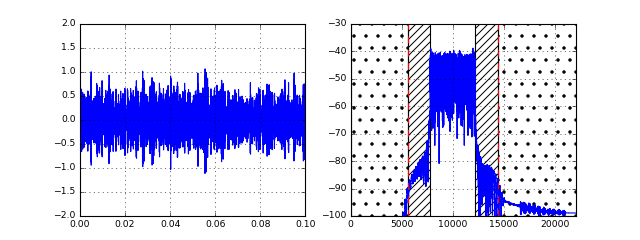Stop received from external


With the OFDM signal, we see a significant portion of power emitted into the dashed region (which should optimally be empty). Let's again compare to the F-OFDM spectrum:

In :
showTXSpectrum(ofdm_narrow, FOFDMTransmitter, AD_DA_filterUseWindow=True, AD_DA_filterLength=9)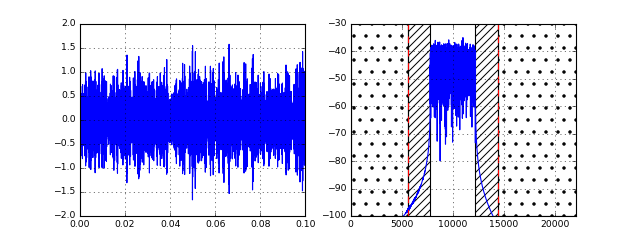Stop received from external


Yes, with the extra filtering operation, the power spectral density within the dashed area significantly reduces, which is exactly what we want.

## Demodulation of an F-OFDM Signal¶

One might argue, it is very simple to transmit a signal with good spectral properties. The challenge is to design a signal that has nice properties but can still be received with a simple receiver. The beauty of F-OFDM is that at the receiver side actually nothing needs to be changed compared to the OFDM receiver. The equalization takes care of the ISI introduced by the subband filter. For the synchronization, we can still use the Schmidl-Cox approach and for channel estimation both block- and comb pilots work. In our implementation, we will resort to block-pilot channel estimation due to its simplicty.

Let us illustrate the demodulation below. We use a similar function compared to the previous notebook, including the control loop for adjusting the synchronization point.

In :
# Source code has been redacted in online version
# Omitting 62 lines of source code


Here's the system configuration we will use for the system. Note the quite narrow allocation to make reception more difficualt:

In :
blockOFDM = OFDM(K=256, Kon=36, qam=4, Npayload=5, CP=64)
blockOFDM.frameLen = (blockOFDM.K + blockOFDM.CP) * (1+1+blockOFDM.Npayload)


First, we define a transmitter object that includes the preamble and pilot block. We simply take the standard OFDM transmitter and add a subsequent subband filter:

In :
class BlockPilotFOFDMTransmitter(BlockPilotRandomDataOFDMTransmitter):
def __init__(self, environment, ofdm):

self._filter = calcFOFDMFilter(ofdm)

def _generateSignal(self):
tx = super()._generateSignal()
return self._filter.filter(tx)

In :
showTXSpectrum(blockOFDM, BlockPilotFOFDMTransmitter, AD_DA_filterUseWindow=True, AD_DA_filterLength=9)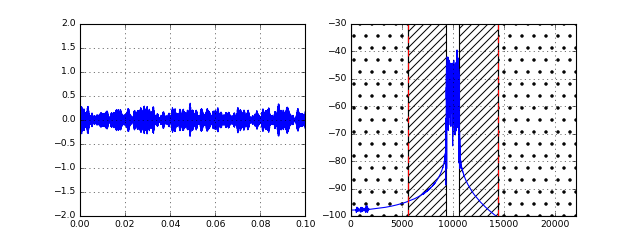Stop received from external


The spectrum looks as we would expect. Now, let's first check our demodulation function using the proven OFDM transmitter and receiver:

In :
# Source code has been redacted in online version
# Omitting 10 lines of source code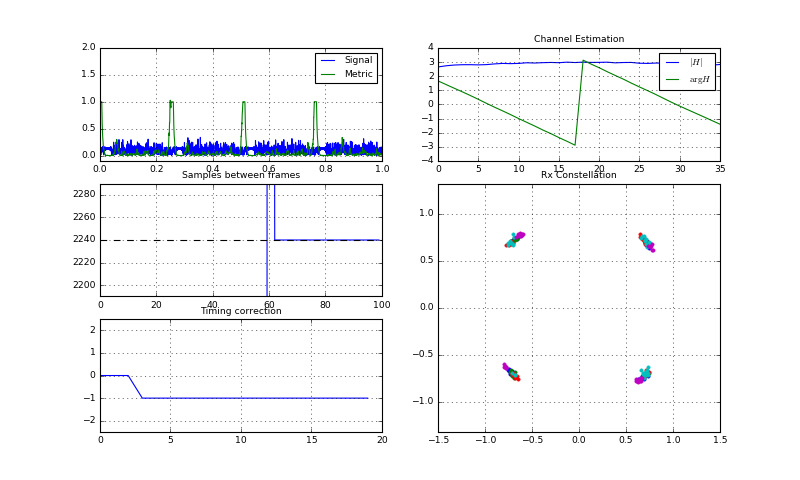Initial sync establibhed
Initial sync establibhed
Stream Stopped 1
Stream Stopped 2


OK great! The synchronization, channel estimation and demodulation work with the narrowly allocated system. Note that when using the audio channel the typical effects of the phase noise (i.e. mainly constellation rotation) are present. For simplicity, we do not implement the full-fledged equalizer here using the comb-pilot scheme.

Now, let's move on and replace the OFDM transmitter with the F-OFDM transmitter. Especially note, that the receiver remains exactly the same (i.e. plain CP-OFDM receiver):

In :
channelFunc = lambda env: SimulatedChannel(env,
ChainedChannelEffects(
[MultipathChannelEffect(taps=[(0,1), (0.005, 0.09)]),
NoiseChannelEffect(0.0001, isComplex=False)]))
channelFunc = AudioChannel  # uncomment to use the Audio channel
runTransmissionWithDemodulation(blockOFDM,
BlockPilotFOFDMTransmitter,  # Using F-OFDM
channelFunc,
lambda env, ofdm: BlockPilotOFDMReceiver(env, ofdm, BlockPilotLSChannelEstimator(ofdm)),
B=441*5*4, Fc=10000, useStreamingMode=True, withTimingCorrection=True)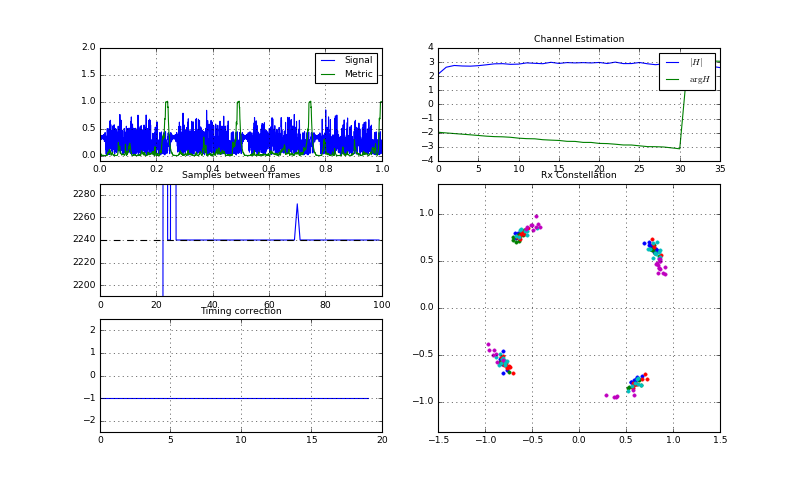Initial sync establibhed
Initial sync establibhed
Underrun!
Initial sync establibhed
Stream Stopped 1
Stream Stopped 2


Yes! After some initial synchronization adjustments, the constellation just looks fine. We emphasize again, that the receiver did not change at all, i.e. any OFDM receiver would in principle be able to receive an F-OFDM signal. This is one major advantage of using F-OFDM for 5G systems, as the know-how and intellectual property about the receiver side can be directly reused. Hence, with F-OFDM we could resolve the problem of the high OOB emission of OFDM, and still be able to use the same receiver.

Let us now look at the second disadvantage of CP-OFDM: Its high PAPR:

## Peak-To-Average Power Ratio¶

The peak-to-average power ratio (PAPR) of a waveform is important as it directly relates to power efficiency of the employed power amplifiers. For example, in the LTE uplink (i.e. the link from your LTE cell phone to the base station) is not using OFDMA due to its high PAPR. Your handheld battery would be vanishing quickly due to the increased power consumption of the power amplifiers. Instead, the LTE uplink employs the SC-FDMA waveform. Below, we will compare PAPR of both techniques:

The PAPR describes the ratio between the peaks of a waveform compared to its mean power. Mathematically, this can be expressed as

$$\text{PAPR} = \frac{\max_t |x(t)|^2}{E[|x(t)|^2]}$$

where $x(t)$ contains the transmit signal that is sent to the power amplifier (PA). The higher the PAPR, the higher needs to be the back-off of the PA. The PA back-off describes, how much higher the instantaneous output power of the PA could be compared to its current mean output power. A high backoff is required to accurately transmit high amplitude peaks in the signal. As the definition of PAPR as above is only a single value it does not tell us a lot. Instead, we look at the distribution of the PAPR over several portions of the signal. This way we get a feeling, how often peaks occur and and how big they actually are. So, usually we consider the complementary cumulative density function (CCDF) of the PAPR given by

 \text{CCDF}_\text{PAPR}(p) = 1-Prob\left[\frac{\max_t |x(t)|^2}{E[|x(t)|^2]}

where $x(t)$ is a portion of the transmit signal sent to the PA.

Let us write a function which evaluates the PAPR for us:

In :
# Source code has been redacted in online version
# Omitting 12 lines of source code


Now, let's look at the (baseband) PAPR for OFDM and SC-FDMA. Note that this PAPR is not exactly accurate, as we omit the DA-conversion and upconversion in this version. See below for a more accurate evaluation.

In :
# Source code has been redacted in online version
# Omitting 5 lines of source code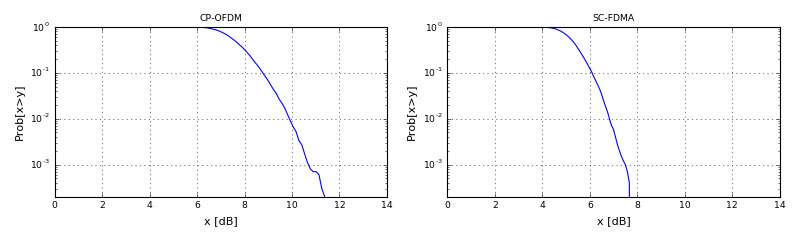There we go, the PAPR of CP-OFDM is roughly 2dB higher than SC-FDMA with the current settings. Play around with the configuration and see the effects. Especially interesting are the QAM and Kon parameters.

Now, that we understood the calculation of PAPR, let's go ahead and run a more sophisticated system. Note that when using the audio channel, the signal is clipped at a value of 1 (which we relate to 0dB). Any amplitude larger than this will not be transmitted correctly. Hence, we have to make sure that we do not often generate amplitudes larger than 1. This is directly related to the PAPR issue.

In the function below, we analyze the transmit signal in three different, but related, metrics:

• The signal's PAPR.
• The signal's CCDf of the amplitude. This metric shows us, how many samples are clipped at the transmission, as any sample above 0dB gets clipped.
• The average power and clipping ratio. This metric shows the mean transmitted power and the resulting clipping ratio.
In :
def showTXPAPRAndSpectrum(txFunc, paprWindowLength, channelFunc=SimulatedChannel):
Fc = 10000
B = 441*5*4
Fs = 44100
env = Environment(samplerate=Fs)

tx = txFunc(env)

chan = PassbandChannel(env, channelFunc=channelFunc, Fc=Fc, B=B, filterLength=9, useWindow=True)

fig = env.figure(figsize=(8,6))
gs = GridSpec(2,6)
showSpectrum = PlotSpectrum(env, windowDuration=0.5, axes=ax, logScale=True, xlim=(0,22500), title='TX Spectrum')
ax.axvline(Fc-B/2, color='black', ls='--')
ax.axvline(Fc+B/2, color='black', ls='--')
showSignal = PlotWaveform(env, windowDuration=0.003, numLines=1, signalTransform=abs, axes=fig.add_subplot(gs[0,:3]), ylim=(0,2), title='TX Signal')
showPower = PlotPowerAndClippingRatio(env, axes=fig.add_subplot(gs[1, :2]), title='TX Power and Clipping Ratio')
showPapr = PlotPAPR(env, windowLength=paprWindowLength, axes=fig.add_subplot(gs[1, 2:4]), title='TX PAPR CCDF')
showCCDF = PlotAmplitudeCCDF(env, axes=fig.add_subplot(gs[1,4:]), title='TX Amplitude CCDF')
fig.tight_layout()

tx.transmitsTo(chan)

chan.transmitsTo(showPower, stream='TxRF')
chan.transmitsTo(showPapr, stream='TxRF')
chan.transmitsTo(showCCDF, stream='TxRF')
chan.transmitsTo(showSignal, stream='TxRF')
chan.transmitsTo(showSpectrum, stream='TxRF')

env.run()


Here's the configuration we use for the evaluation:

In :
papr_ofdm = OFDM(K=256, Kon=201, CP=16, Npayload=2, qam=4)


In the first simulation we evaluate the plain CP-OFDM system. The gain is already set such that the clipping ratio is roughly 1%.

In :
showTXPAPRAndSpectrum(lambda env: OFDMTransmitter(env, papr_ofdm, gain=1), paprWindowLength=papr_ofdm.K+papr_ofdm.CP, channelFunc=AudioChannel)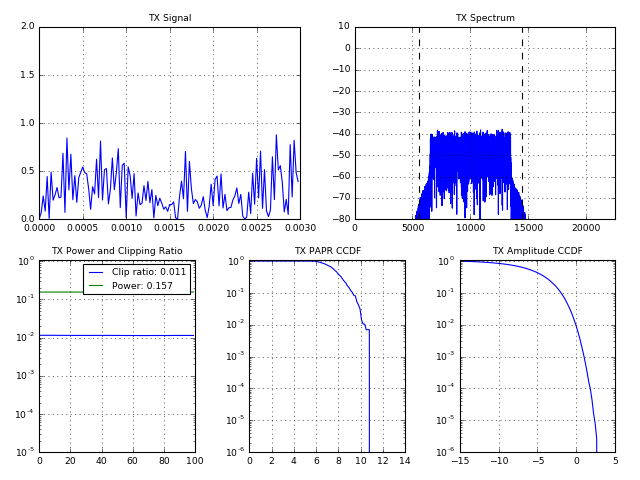Underrun!

showTXPAPRAndSpectrum(lambda env: SCFDMATransmitter(env, papr_ofdm, gain=1.23), paprWindowLength=papr_ofdm.K+papr_ofdm.CP, channelFunc=AudioChannel)